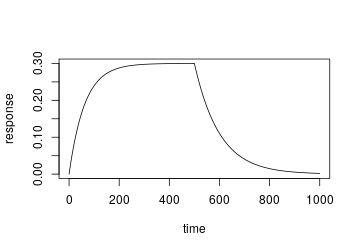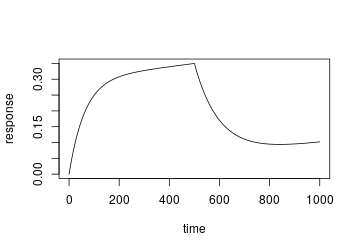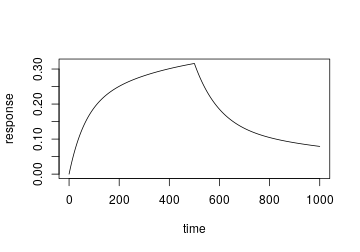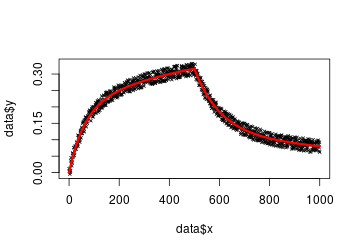When collecting protein-ligand binding data using a technique such as Biolayer Interferometry (BLI) or Surface Plasmon Resonance (SPR), it is useful to simulate binding curves to help optimise your experiments. After initial binding parameters are known, binding curves can be simulated and parameters such as: analyte concentration, time of association, dissociation etc. can be varied. The models within this package may also be used to fit a curve to measured binding data using a non-linear regression.

Currently, two binding models are included with this package:

• 1:1 binding
• 2:1 heterogeneous binding.

## 1:1 Binding

``````library(pbm)
time <- seq(0,1000)
response <- binding1to1(time,500,6e-7,10000,0.01,0.8)
plot(time, response, type="l")
``````### Optional drift parameter

``````library(pbm)
time <- seq(0,1000)
response <- binding1to1(time,500,6e-7,10000,0.01,0.8, drift = 1e-04)
#> Warning in binding1to1(time, 500, 6e-07, 10000, 0.01, 0.8, drift = 1e-04):
#> Drift parameter set
plot(time, response, type="l")
``````## 2:1 Binding

``````library(pbm)
time <- seq(0,1000)
response <- binding2to1(time,500,6e-7,10000,0.01,0.5,2500,0.001,0.3)
plot(time, response, type="l")
``````## Non-linear Regression

``````library(pbm)

# Generate example binding data with noise
time <- seq(0,1000)
response <- binding2to1(time,500,6e-7,10000,0.01,0.5,2500,0.001,0.3)
noisyresponse <- jitter(response,amount=0.02)
data <- data.frame(time, noisyresponse)
names(data) <- c("x","y")

# Fit a nlm to binding data
startingvalues <- list(kon1=70000,koff1=0.01,rmax1=0.3,kon2=9000,koff2=0.004,rmax2=0.3)
fit <- nls(y ~ binding2to1(x,500,6e-7,kon1,koff1,rmax1,kon2,koff2,rmax2),
data=data,
start=startingvalues)

# Plot the fitted model
plot(data\$x, data\$y, type="p", pch=4, cex=.5)
par(col="red",lwd=3)
lines(data\$x,predict(fit, list(x=data\$x)))
``````Parameters predicted from fitted model:

kon1 koff1 rmax1 kon2 koff2 rmax2
11218.59 0.0098071 0.4721689 1792.759 0.0009171 0.3639649